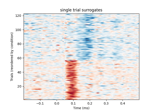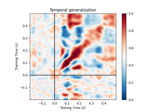# mne.decoding.EMS¶

class mne.decoding.EMS[source]

Transformer to compute event-matched spatial filters.

This version of EMS 1 operates on the entire time course. No time window needs to be specified. The result is a spatial filter at each time point and a corresponding time course. Intuitively, the result gives the similarity between the filter at each time point and the data vector (sensors) at that time point.

Note

EMS only works for binary classification.

References

1

Aaron Schurger, Sebastien Marti, and Stanislas Dehaene. Reducing multi-sensor data to a single time course that reveals experimental effects. BMC Neuroscience, 2013. doi:10.1186/1471-2202-14-122.

Attributes
filters_`ndarray`, shape (n_channels, n_times)

The set of spatial filters.

classes_`ndarray`, shape (n_classes,)

The target classes.

Methods

 `__hash__`(/) Return hash(self). `fit`(X, y) Fit the spatial filters. `fit_transform`(X[, y]) Fit to data, then transform it. `get_params`([deep]) Get the estimator params. `set_params`(**params) Set parameters (mimics sklearn API). Transform the data by the spatial filters.
fit(X, y)[source]

Fit the spatial filters.

Parameters
X`array`, shape (n_epochs, n_channels, n_times)

The training data.

y`array` of `int`, shape (n_epochs)

The target classes.

Returns
selfinstance of `EMS`

Returns self.

Examples using `fit`:fit_transform(X, y=None, **fit_params)[source]

Fit to data, then transform it.

Fits transformer to X and y with optional parameters fit_params and returns a transformed version of X.

Parameters
X`array`, shape (n_samples, n_features)

Training set.

y`array`, shape (n_samples,)

Target values.

**fit_params`dict`

Additional fitting parameters passed to `self.fit`.

Returns
X_new`array`, shape (n_samples, n_features_new)

Transformed array.

get_params(deep=True)[source]

Get the estimator params.

Parameters
deepbool

Deep.

set_params(**params)[source]

Set parameters (mimics sklearn API).

Parameters
**params`dict`

Extra parameters.

Returns
instobject

The instance.

transform(X)[source]

Transform the data by the spatial filters.

Parameters
X`array`, shape (n_epochs, n_channels, n_times)

The input data.

Returns
X`array`, shape (n_epochs, n_times)

The input data transformed by the spatial filters.

Examples using `transform`:## Examples using `mne.decoding.EMS`¶